×
INTELLIGENT WORK FORUMS
FOR ENGINEERING PROFESSIONALS

Are you an
Engineering professional?
Join Eng-Tips Forums!
• Talk With Other Members
• Be Notified Of Responses
• Keyword Search
Favorite Forums
• Automated Signatures
• Best Of All, It's Free!

*Eng-Tips's functionality depends on members receiving e-mail. By joining you are opting in to receive e-mail.

#### Posting Guidelines

Promoting, selling, recruiting, coursework and thesis posting is forbidden.

# Structure Design Using Slope-Deflection Equations and Matlab

## Structure Design Using Slope-Deflection Equations and Matlab

(OP)
Hi there, I was wondering if someone could help me.

I am designing a simple structure. I have attached a diagram and I am using the slope-deflection equations and using Matlab. Unfortunately I am getting strange results that I think are wrong. For the rotations at the joints I am getting zero which cannot be correct.

Here is my Matlab code:

clear
clc

% data

E = 2e+08;
I = 0.002;
EI = E.*I;
L1 = 5;
L2 = sqrt(5.^2 + 5.^2);
q = 100;
FEM1 = q.*L1.^2./12;
FEM2 = -FEM1;

% Symbolic mathematics

syms X1 X2 X3 X4 X5 X6 X7 X8 X9 X10

X1 = 0; X3 = 0; X5 = 0; X7 = 0;
X2 = -X8;
X4 = -X6;
X9 = -X10;

M1i = 4.*EI./L1.*X1 + 2.*EI./L1.*X2;
M1j = 2.*EI./L1.*X1 + 4.*EI./L1.*X2;

M2i = 4.*EI./L1.*X3 + 2.*EI./L1.*X4;
M2j = 2.*EI./L1.*X3 + 4.*EI./L1.*X4;

M3i = 4.*EI./L1.*X5 + 2.*EI./L1.*X6;
M3j = 2.*EI./L1.*X5 + 4.*EI./L1.*X6;

M4i = 4.*EI./L1.*X7 + 2.*EI./L1.*X8;
M4j = 2.*EI./L1.*X7 + 4.*EI./L1.*X8;

M5i = 4.*EI./L1.*X2 + 2.*EI./L1.*X4;
M5j = 2.*EI./L1.*X2 + 4.*EI./L1.*X4;

M6i = 4.*EI./L1.*X4 + 2.*EI./L1.*X6;
M6j = 2.*EI./L1.*X4 + 4.*EI./L1.*X6;

M7i = 4.*EI./L1.*X6 + 2.*EI./L1.*X8;
M7j = 2.*EI./L1.*X6 + 4.*EI./L1.*X8;

M8i = 4.*EI./L2.*X2 + 2.*EI./L2.*X9;
M8j = 2.*EI./L2.*X2 + 4.*EI./L2.*X9;

M9i = 4.*EI./L1.*X9 + 2.*EI./L1.*X10 + FEM1;
M9j = 2.*EI./L1.*X9 + 4.*EI./L1.*X10 + FEM2;

M10i = 4.*EI./L2.*X10 + 2.*EI./L2.*X8;
M10j = 2.*EI./L2.*X10 + 4.*EI./L2.*X8;

M11i = 4.*EI./L1.*X4 + 2.*EI./L1.*X9;
M11j = 2.*EI./L1.*X4 + 4.*EI./L1.*X9;

M12i = 4.*EI./L1.*X6 + 2.*EI./L1.*X10;
M12j = 2.*EI./L1.*X6 + 4.*EI./L1.*X10;

% Solving

Joint2 = M1j + M5i + M8i == 0

Joint4 = M2j + M5j + M11i + M6i == 0

Joint6 = M3i + M6j + M12i + M7i == 0

Joint8 = M4j + M7j + M10j == 0

Joint9 = M8j + M11j + M9i == 0

Joint10 = M9j + M10i + M12j == 0

I am hoping someone here knows how to use Matlab and is familiar with slope=deflection equations that can help me.

Kind regards

### RE: Structure Design Using Slope-Deflection Equations and Matlab

I haven't used solve for years but you have only initialised 4 of the Xs.

You need to list all the relevant Xs in each solve line, not just X6 , and I am fairly convinced your last line won't work.

Cheers

Greg Locock

New here? Try reading these, they might help FAQ731-376: Eng-Tips.com Forum Policies http://eng-tips.com/market.cfm?

### RE: Structure Design Using Slope-Deflection Equations and Matlab

(OP)
Hi Greg, could you elaborate a bit more? Maybe could you copy and paste my Matlab code in yours and analyse it?

### RE: Structure Design Using Slope-Deflection Equations and Matlab

(OP)
Is there any structural engineer here that can help?

### RE: Structure Design Using Slope-Deflection Equations and Matlab

Well, I could possibly help with the structural part, but I am not familiar with Matlab, so I don't understand all of your equations.

Since the structure and the load are symmetrical, you could write slope-deflection equations for only half the joints. In your case, that would be joints 1, 2, 3, 4 and 9. Of course, if you load the structure unsymmetrically, you will need to write equations for every joint.

The slope deflection method, for any member spanning from A to B has two equations which are easily verified, namely:

MAB = MFAB + 2EI/L *(-2θA - θB)

MBA = MFBA + 2EI/L *(-2θB - θA)

EDIT: There are only three unknown rotations. They occur at nodes 2, 4 and 9 (rotation at nodes 1 and 3 is zero), so it should result in three simultaneous equations which should be soluble by hand methods. From that, the moments can be determined at all nodes.

BA

### RE: Structure Design Using Slope-Deflection Equations and Matlab

I go with BAretired. MATLAB is basically a matrix program, have them in a collective matrix and use inverse to find rotations. I also see "syms", did you use "eval" to evaluate those symbols? I haven't used MATLAB in a while so it could be I am missing something..

1. MAB = MFAB + 2EI/L *(-2θA - θB);
2. MBA = MFBA + 2EI/L *(-2θB - θA);
Rewriting 1. MAB = MFAB - 4EI/L*θA -2EI/L*θB
Rewriting 2. MBA = MFBA - 4EI/L*θB -2EI/L*θA

Matrix becomes
[M] = [FEM - K][θ]
MAB = [MFAB - 4EI/L - 2EI/L][θA]
MBA = [MFBA - 2EI/L - 4EI/L][θB]
since this expression is 'linear' use "\" not "inv" function. You can try "inv", I think that takes lots of computation time. Like I said, it has been a while I used MATLAb but I hope this helps

### RE: Structure Design Using Slope-Deflection Equations and Matlab

Alright, I think I see how you did yours, you used the "solve" function so it's basically the same concept. I would have to run your script and review the it later...let me know in the mean time if you find a fix or where the error was.

### RE: Structure Design Using Slope-Deflection Equations and Matlab

(OP)
Hi NicOkai, thanks for your help. I still haven't figured what I am doing wrong. If you could do that, that would be great.

Have you gone through my slope-deflection equations at all? Because I'm not sure if the error is with my calculations or something to do with my Matlab code. I am using Matlab simply to reduce number crunching and make the calculations easier on myself. I downloaded the symbolic toolbox for Matlab so I could use symbolic mathematics.

Thanks again.

### RE: Structure Design Using Slope-Deflection Equations and Matlab

(OP)
And I have used this format for my slope-deflection equations.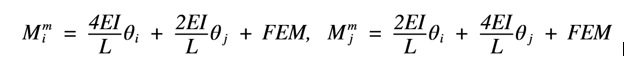It Matlab I have replaced the theta1, theta2, theta3 etc... with X1, X2, X3 etc...

### RE: Structure Design Using Slope-Deflection Equations and Matlab

The only difference I see is with the sign convention. In your formula, the theta values are positive when counterclockwise, but it shouldn't make any difference to the magnitude. For example, the FEM at Joint 9 is clockwise. With your equations, θ9 would be negative, but the magnitude would be the same.

BA

### RE: Structure Design Using Slope-Deflection Equations and Matlab

Hello Tygra, I got a little busy and couldn't run your script before. I will rub your script and go through but if you can, can you kindly try solving a simply-supported beam with the same script? Modify parameters to fit conditions and let's see the results we get.

Joint6 = M3i + M6j + M12i + M7i == 0 should be Joint6 = M3j + M6j + M12i + M7i == 0

### RE: Structure Design Using Slope-Deflection Equations and Matlab

(OP)
Here, NicOkia

clear
clc
close all

L = 5;
q = 100
FEM1 = q.*L.^2./12;
EI = 400000;
FEM2 = -FEM1;

syms X1 X2

M1i = 4.*EI./L.*X1 + 2.*EI./L.*X2 + FEM1 == 0;
M1j = 2.*EI./L.*X1 + 4.*EI./L.*X2 + FEM2 == 0;

theta1 = -theta2

PS: thanks BA retired for your help also!

### RE: Structure Design Using Slope-Deflection Equations and Matlab

(OP)
Hi NicOkia,

Unfortunately, changing the equation using the j component still gives a result of X6,X8,X10 = 0. How have you found the code in your Matlab?

### RE: Structure Design Using Slope-Deflection Equations and Matlab

@Tygra-1983

Try deleting the following lines from your code. The program should find all rotations without these lines.

X2 = -X8;
X4 = -X6;
X9 = -X10;

BA

### RE: Structure Design Using Slope-Deflection Equations and Matlab

(OP)
Unfortunately, I'm still get the rotations to be zero BAretired! Even with that change.

### RE: Structure Design Using Slope-Deflection Equations and Matlab

Tygra,

Sorry, I failed to save the MATLAB file I was working on initially and was busy as well. I have a reminder set for me to go through your scripts again but I have scribbled something down to help us check. I would like you to make "E = 2e8", "I = 0.002", "L1 = 5", "L2 = sqrt(5.^2 + 5.^2)" as comments. Also instead of having FEM1 as an equation, just input 208.333. Run the script up to "Joint10 = M9j + M10i + M12j == 0".

I think the problem will come from "Answer1 = solve(Joint2,X6) Answer2 = solve(Joint4,X6) Answer3 = solve(Joint6,X6) Answer4 = solve(Joint8,X6)" so I want us to be sure. What I am seeing is, MATLAB is solving equation "Answer1 = solve(Joint2,X6)" for theta 6 but there's no theta 6 in Joint 2? For a system of equations, solutions are also dependent on other equations but I don;t see it in the MATLAB (from the way I see it). Lets say you have 3 unknowns and 3 equations, you need to solve them "simultaneously" but that's not done here. When you're done going through my attachment after making those changes I mentioned up there, try rewriting the system equations for the joints.

### RE: Structure Design Using Slope-Deflection Equations and Matlab

(OP)
NicOkai, I think it is sorted. Firstly, I went straight ahead and changed Answer1 Answer2 etc... because that made a lot of sense. And now I have values that look correct.

I will paste the entire script and command window:

% data

E = 2e+08;
I = 0.002;
EI = E.*I;
L1 = 5;
L2 = sqrt(5.^2 + 5.^2);
q = 100;
FEM1 = q.*L1.^2./12;
FEM2 = -FEM1;

% Symbolic mathematics

syms X1 X2 X3 X4 X5 X6 X7 X8 X9 X10

X1 = 0; X3 = 0; X5 = 0; X7 = 0;

M1i = 4.*EI./L1.*X1 + 2.*EI./L1.*X2;
M1j = 2.*EI./L1.*X1 + 4.*EI./L1.*X2;

M2i = 4.*EI./L1.*X3 + 2.*EI./L1.*X4;
M2j = 2.*EI./L1.*X3 + 4.*EI./L1.*X4;

M3i = 4.*EI./L1.*X5 + 2.*EI./L1.*X6;
M3j = 2.*EI./L1.*X5 + 4.*EI./L1.*X6;

M4i = 4.*EI./L1.*X7 + 2.*EI./L1.*X8;
M4j = 2.*EI./L1.*X7 + 4.*EI./L1.*X8;

M5i = 4.*EI./L1.*X2 + 2.*EI./L1.*X4;
M5j = 2.*EI./L1.*X2 + 4.*EI./L1.*X4;

M6i = 4.*EI./L1.*X4 + 2.*EI./L1.*X6;
M6j = 2.*EI./L1.*X4 + 4.*EI./L1.*X6;

M7i = 4.*EI./L1.*X6 + 2.*EI./L1.*X8;
M7j = 2.*EI./L1.*X6 + 4.*EI./L1.*X8;

M8i = 4.*EI./L2.*X2 + 2.*EI./L2.*X9;
M8j = 2.*EI./L2.*X2 + 4.*EI./L2.*X9;

M9i = 4.*EI./L1.*X9 + 2.*EI./L1.*X10 + FEM1;
M9j = 2.*EI./L1.*X9 + 4.*EI./L1.*X10 + FEM2;

M10i = 4.*EI./L2.*X10 + 2.*EI./L2.*X8;
M10j = 2.*EI./L2.*X10 + 4.*EI./L2.*X8;

M11i = 4.*EI./L1.*X4 + 2.*EI./L1.*X9;
M11j = 2.*EI./L1.*X4 + 4.*EI./L1.*X9;

M12i = 4.*EI./L1.*X6 + 2.*EI./L1.*X10;
M12j = 2.*EI./L1.*X6 + 4.*EI./L1.*X10;

% Solving

Joint2 = vpa(M1j + M5i + M8i == 0,3)

Joint4 = vpa(M2j + M5j + M11i + M6i == 0,3)

Joint6 = vpa(M3j + M6j + M12i + M7i == 0,3)

Joint8 = vpa(M4j + M7j + M10j == 0,3)

Joint9 = vpa(M8j + M11j + M9i == 0,3)

Joint10 = vpa(M9j + M10i + M12j == 0,3)

Command Window:

Joint2 =

8.66e+5*X2 + 1.6e+5*X4 + 1.13e+5*X9 == 0.0

Joint4 =

1.6e+5*X2 + 1.28e+6*X4 + 1.6e+5*X6 + 1.6e+5*X9 == 0.0

Joint6 =

1.6e+5*X4 + 1.28e+6*X6 + 1.6e+5*X8 + 1.6e+5*X10 == 0.0

Joint8 =

1.6e+5*X6 + 8.66e+5*X8 + 1.13e+5*X10 == 0.0

Joint9 =

1.13e+5*X2 + 1.6e+5*X4 + 8.66e+5*X9 + 1.6e+5*X10 + 208.0 == 0.0

Joint10 =

1.6e+5*X6 + 1.13e+5*X8 + 1.6e+5*X9 + 8.66e+5*X10 - 208.0 == 0.0

- 0.1847*X4 - 0.1306*X9

- 0.125*X2 - 0.125*X6 - 0.125*X9

- 0.125*X4 - 0.125*X8 - 0.125*X10

- 0.1847*X6 - 0.1306*X10

- 0.1306*X2 - 0.1847*X4 - 0.1847*X10 - 0.00024049

0.00024049 - 0.1306*X8 - 0.1847*X9 - 0.1847*X6

theta =

struct with fields:

X2: -0.00076274275732299026156747397808267
X4: -0.00031593839468454238506193227735856
X6: 0.00031593839468454238506193227735856
X8: 0.00076274275732299026156747397808267
X9: 0.00044680436263844787650554170072411
X10: -0.00044680436263844787650554170072411

What do you think??

Thanks so much for all your time an effort.

### RE: Structure Design Using Slope-Deflection Equations and Matlab

Congratulations Tygra_1983! Perseverance paid off.

BA

### RE: Structure Design Using Slope-Deflection Equations and Matlab

That's good. You're getting results now. Let's validate those number now, plug them back in your joint equations and see if you get zeroes for the summation of moments. If yes, then you're good!

### RE: Structure Design Using Slope-Deflection Equations and Matlab

(OP)
No, unfortunately, I am not getting zero for the sum of the moments about the joints, NicOKai: Dam! I think we are better off than we were though - @BAretired.

### RE: Structure Design Using Slope-Deflection Equations and Matlab

Sorry to hear that. I found x2, x4 and x9 to be as follows, but I used relative EI/L, not the actual values:
x2 -5.294935592
x4 -6.311284333
x9 49.47392592

For the typical member, I considered K = 2EI/L to be 1.0, but for member 8, K would be 0.70711

On that basis, I find the following moments:

M2-1 = 0 - 2*x2 = 10.6kN=m
M2-4 = 0 - 2*x2 - x4 = 16.9
M2-9 = 0 - 0.7071(-2*x2 - x9) = -27.4kN-m ------- Sum = 0 (check)

M4-2 = 0 - (2*x4 +x2) = 17.9
M4-3 = 0 - 2*x4 = 12.6
M4-6 = 0 - (2*x4 +x6) = -x4 = 6.3
M4-9 = 0 - (2*x4 +x9) = -36.85 ------- Sum = 0 (check)

M9-2 = 0 - 0.70711(2*x9 + x2) = -66.22
M9-4 = 0 - (2*x9 + x4) = -92.64
M9-10 = 208.33 - (2*x9 + x10) = 208.33 - x9 = 158.85kN-m ------- Sum = 0 (check)

The sum of moments at Joints 2, 4 and 9 is zero as expected.

BA

### RE: Structure Design Using Slope-Deflection Equations and Matlab

@Tygra,

I did not use your EI values, so had not noticed that your values seem a bit inconsistent. If actual rotations and deflections are required, the correct values are needed.

The following are the values you used:

E = 2e+08; a value of 200,000,000 (not the usual units); E for steel is normally taken as 200,000 MPa, which is the same as 200,000 N/mm2
I = 0.002; that seems very small. It should be given in consistent units.
EI = E.*I;
L1 = 5; this term is in meters.
L2 = sqrt(5.^2 + 5.^2);
q = 100; this term is in kN/m
FEM1 = q.*L1.^2./12; this results in units of kN-m
FEM2 = -FEM1;
It is advisable to maintain a consistent set of units throughout all calculations. If you don't, you tend to get very strange results. As mentioned earlier, for this problem, relative stiffness values are all that is required.

BA

### RE: Structure Design Using Slope-Deflection Equations and Matlab

(OP)
I changed the units to be consistent. Thanks for pointing that out BAretired. However, still getting wrong results. I appreciate your method BAretired, but I would really like to crack this the way I have originally set out. When this problem is solved I would like to move on to even bigger structures, as I think this would be good for my career as civil engineer. saying this I have no idea what the error is in this problem. I am confused because on smaller problems I am getting correct answers. For example, this: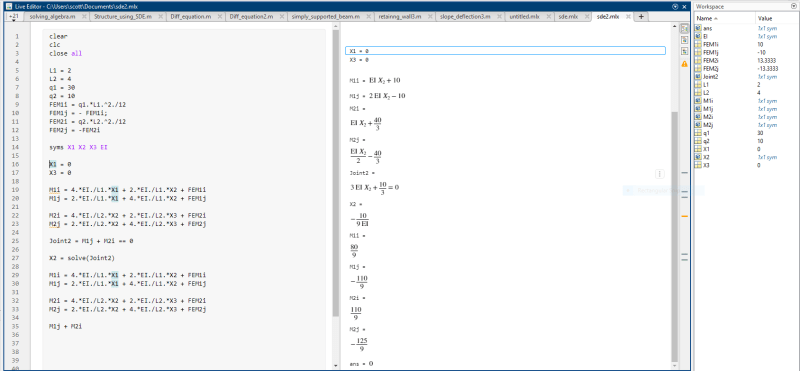As you can see this is correct

### RE: Structure Design Using Slope-Deflection Equations and Matlab

It appears to me that you have some errors in your joint equations. The sketch below shows the joint coefficients you should be using. They include the length difference for the diagonal members.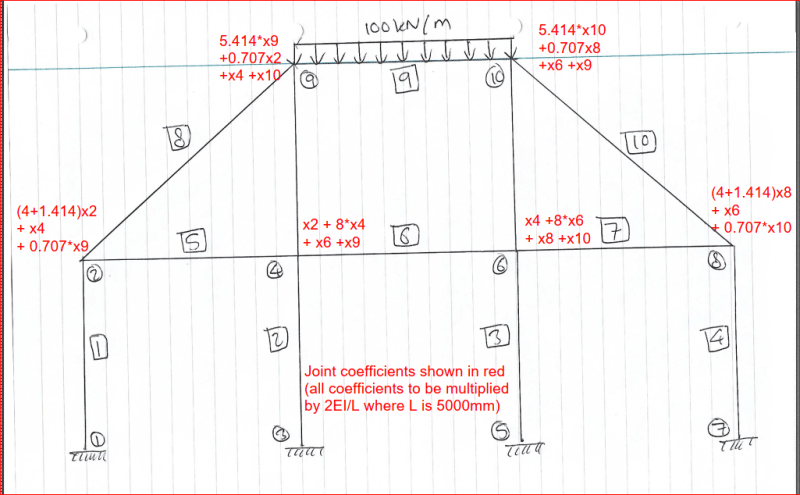@Tygra,

After writing the above, I was unable to find the inverse of the matrix. I don't know what is wrong.

Update: Found the inverse, but the answers can't be right because x2, x4 and x9 do not equal -x8, -x6 and -x10 respectively, which they must because of symmetry. Looks like we'll both have to sleep on it.

Update #2: Found the error. Made a mistake in typing one of the cells. The answers agree with the results I found using only three nodes.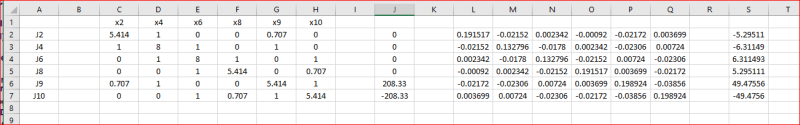Rotations x2 through x10 are shown in column S of the table above. To get the correct rotation, multiply all values by 2EI/L.

BA

### RE: Structure Design Using Slope-Deflection Equations and Matlab

(OP)
BAretrired, that is amazing what you have done. However, could your point out what error I made in my joint equations? This will be good for learning.

### RE: Structure Design Using Slope-Deflection Equations and Matlab

#### Quote (Tygra)

BAretrired, that is amazing what you have done. However, could your point out what error I made in my joint equations? This will be good for learning.

I believe I was mistaken about an error in your joint equations. So far as I can see, they are all okay. I am attaching the results of both sets of calculations (see below). In both sets of results, the original joint matrix is from columns C to H. Fixed end moments are in column J. The inverse matrices are from columns L to Q and the final values of rotations are in column S.

In both cases, x2 = -x8; x4 = -x6 and x9 = -x10.

The sum of Joint moments is zero for both results below. If you are getting different results from those that I show, the problem may lie in a misunderstanding or misapplication of your software.

BA results on Rows 2 to 7:
Tygra results on Rows 9 to 14: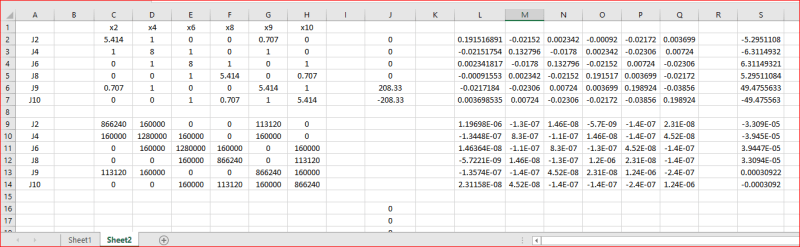Conclusion: there is nothing wrong with the equations in either set above. In both cases, the sum of moments at every joint is zero.

BA

### RE: Structure Design Using Slope-Deflection Equations and Matlab

(OP)
I think it is a misunderstanding or a misapplication of my software (Matlab). However, I am not sure what route to go down though. I was thinking of making a matrix for X2,X4,X6,X8,X9,X10 values, but that is quite tedious. What do you think NicOkai?

Edit: just caught your edited post BAretired. Hoping you can save me from this torment tomorrow lol.

Edit2: I think we need someone that is exceptional in Matlab too.

### RE: Structure Design Using Slope-Deflection Equations and Matlab

Tygra,

So the way I described it is similar to how BAretired solved it.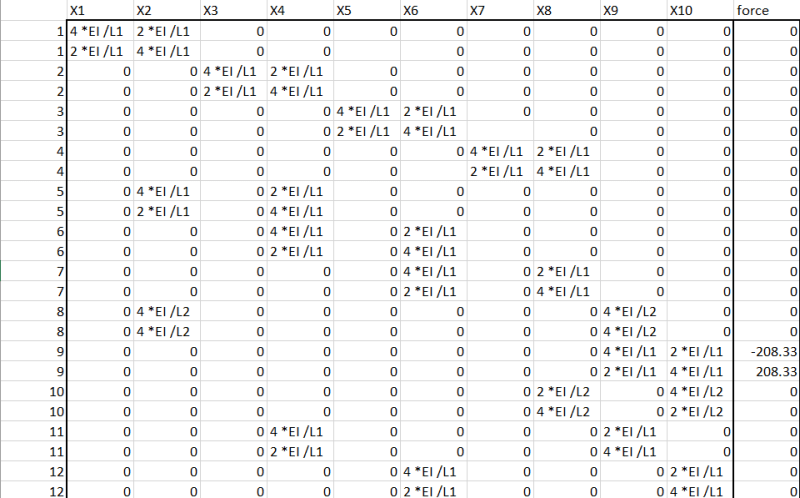FEM = [stiffness][rotation]
rotation = FEM\stiffness. I have attached the excel, sheet2 is the numerical expression. Try that and see if it works (kindly verify my units of E and I in the spreadsheet. Is this due tomorrow or it's a personal project? (I have an ideal but that we don't have time for that now, it involves writing a whole different script)

EDIT
If you break it down to Joint 2, Joint 4, Joint 6, Joint 8, Joint 9, and Joint 10 you should get the same matrix as BAretired.

### RE: Structure Design Using Slope-Deflection Equations and Matlab

#### Quote (Tygra)

I think it is a misunderstanding or a misapplication of my software (Matlab)
I'd say you understand but misapplication. The script has been written in a fixed manner, meaning, you can't run it once to solve the problem. It is going to be tedious to make the matrix. To simplify things for yourself, do it like BAtired. Get you equations, go to excel or another matlab tab, run them and get the values and solve the inverse.

#### Quote (Tygra)

When this problem is solved I would like to move on to even bigger structures, as I think this would be good for my career as civil engineer.
We did something similar using the stiffness matrix back in school but I can't share the script since I wasn't the only one who worked on it but I can share the idea later with you. I tried getting my friend to give me access to his MATLAB account since I no longer have the license but couldn't reach him. I'm planning to learn Python since it is free and try developing scripts for my designs as well.

I am really sorry I couldn't be of help at the moment. All I can say is BAretired got it correct in Excel.

### RE: Structure Design Using Slope-Deflection Equations and Matlab

(OP)

#### Quote:

Is this due tomorrow or it's a personal project?

In November there will be a coursework where we have to design a steel-framed building. The lecturer said we will need our knowledge from the previous modules of structural and stress analysis in year one and structural analysis in year 2. For this reason, I have been pratising with slope-defection equations that I learnt in structural analysis to prepare myself for the coursework. I might be wrong, but I think the structure for the coursework might be more complicated than the example we have been working on.

#### Quote:

We did something similar using the stiffness matrix back in school but I can't share the script since I wasn't the only one who worked on it but I can share the idea later with you. I tried getting my friend to give me access to his MATLAB account since I no longer have the license but couldn't reach him. I'm planning to learn Python since it is free and try developing scripts for my designs as well.

I am really sorry I couldn't be of help at the moment. All I can say is BAretired got it correct in Excel.

That is absolutely fine. I thank you and BAretired for your patience and all your help thus far. I have decided to adopt your method, but I thought I would have a go at solving a smaller structure. However, I am not getting the correct rotations. Could you check my workings to see where I have gone wrong, NicOkai?

Here is the structure with its answers.

This is my Excel matrix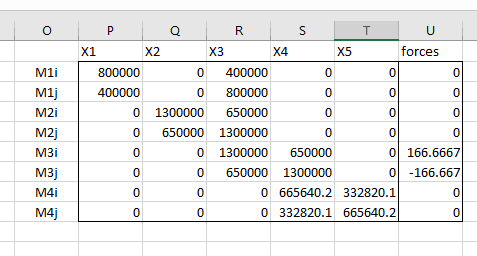This is the Matlab code with the rotations I am getting using.

A = [800000 0 400000 0 0
400000 0 800000 0 0
0 1300000 650000 0 0
0 650000 1300000 0 0
0 0 1300000 650000 0
0 0 650000 1300000 0
0 0 0 665640.2355 332820.1177
0 0 0 332820.1177 665640.2355]

B = [0
0
0
0
166.6666667
-166.6666667
0
0
]

Y = pinv(A)*B

1.0e-03 *

-0.0779
-0.0779
0.0974
-0.1180
0.0944

### RE: Structure Design Using Slope-Deflection Equations and Matlab

Hint:

You have to solve two equations, one for Joint 3 and one for Joint 4. The other joints are all fixed.

BA

### RE: Structure Design Using Slope-Deflection Equations and Matlab

#### Quote (BAretired)

You have to solve two equations, one for Joint 3 and one for Joint 4. The other joints are all fixed.

Only Joint 3 and 4 can rotate. Also I think MATLAB "pinv" gives "different" solution" as it's a pseudo inverse. Try using "pinv", "inv" and "/" to solve an inverse of matrix you know the solution and watch the results you get.

### RE: Structure Design Using Slope-Deflection Equations and Matlab

(OP)
Still getting Incorrect results guys.

m1i = 0.8e+6, m1j = m1i./2
m2i = 1.3e+6, m2j = m2i./2
m4i = (2e+8.*0.006)./sqrt(4.^2+6.^2), m4j = m4i./2
FEM1 = 166.667
FEM2 = -166.667

syms X1 X2 X3 X4 X5

X1 = 0; X2 = 0; X5 = 0

M1i = m1i.*X1 + m1j.*X3
M1j = m1j.*X1 + m1i.*X3

M2i = m2i.*X2 + m2j.*X3
M2j = m2j.*X2 + m2i.*X3

M3i = vpa(m2i.*X3 + m2j.*X4 + FEM1,5)
M3j = vpa(m2j.*X3 + m2i.*X4 + FEM2,5)

M4i = vpa(m4i.*X4 + m4j.*X5,5)
M4j = vpa(m4j.*X4 + m4i.*X5,5)

joint3 = vpa(M1j + M2j + M3i,5)
Joint4 = vpa(M3j + M4i,5)

y1 = equationsToMatrix(joint3)
y2 = equationsToMatrix(Joint4)

A = [y1; y2]
B = [FEM1; FEM2]

Theta = inv(A)*B

X3 = Theta(1) = 0.000077298482151272739116422631226081
X4 = Theta(2) = 0.00014791975279127278677572495087085

3.4e+6.*X3 + 650000.*X4 + 166.67 = 333.33700000000000159161572810262

### RE: Structure Design Using Slope-Deflection Equations and Matlab

I'm not sure how you arrived at those values for X3 and X4, but my calcs are shown below. I have used relative stiffness values instead of the actual values, because all those zeros tend to confuse me, so the rotations are not identical to those shown in Answers, item (c), in fact, they differ by a factor of 10^6.

Column 1:
4EI/L = 0.8*10^6;
k1 = 0.8
Beam 2, 3:
4EI/L = 1.3*10^6;
k2 = k3 = 1.3
Beam 4:
4EI/L = 2*10^8*0.006/5 = 665,640
k4 = 0.6656

Slope-deflection equations
Fixed end moment = 125(4^2)/12 = 166.67kN-m

Joint 3: θ3(k1+k2+k3) + θ4(k3/2) = 166.67
(0.8+1.3+1.3)θ3 + (1.3/2)θ4 = 166.67
θ3*3.4 + θ4*0.65 = 166.67-----------------------(1)
Joint 4: θ3(k3/2) + θ4(k3+k4) = -166.67
θ3*0.65 + θ4*1.9656 = -166.67------------------(2)
(2) times (3.4/0.65)
θ3*3.40 + θ4*10.282= -871.81------------------(3)
(1) minus (3)
-9.632θ4 = 1038.48;
θ4 = -107.82
From Equation (1),
θ3 = [166.67 - (-107.82*0.65)]/3.40 = 69.63

BA

### RE: Structure Design Using Slope-Deflection Equations and Matlab

For the last parts to the question, see below:

Question (d)
Moment in Beam 2 is -0.65*θ3 = -45.26kN-m and -1.3*θ3 = -90.52kN-m left and right respectively.

Question (e)
member 3: Msimple = wl^2/8 = 250kN-m
m3 left = 166.67 - k3(θ3 + θ4/2) = 146.23
m3 right = -166.67 - k3(θ3/2 + θ4) = -71.76
m3 midspan = 250 - (141.0 + 94.90)/2 = 141.0kN-m

BA

@Tygra,

BA

### RE: Structure Design Using Slope-Deflection Equations and Matlab

(OP)
Hi BAretired,

Yes, I have solved both problems now, although the smaller problem does have me scratching my head a bit. This is because I have attained the answers that in the handout (the yellow sheet I previously attached), but strangely my joint equations doesn't equal zero. See the image below: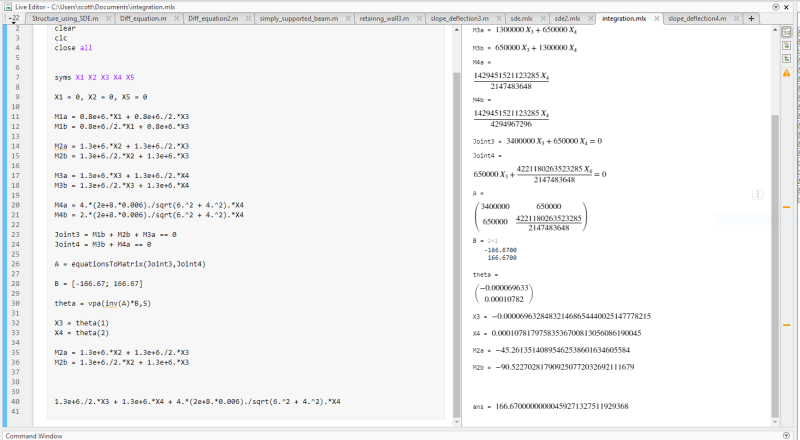For the larger problem my joint equations don't equal zero exactly, but they are a very small number close to zero - like 7.3e-39. Do you think this could well be the correct answers? The rotations match the symmetrical geometry of the structure too.

### RE: Structure Design Using Slope-Deflection Equations and Matlab

Sounds good to me! 7.9e-39 is about as close to zero as you will get with a computer. Congratulations!

BA

### RE: Structure Design Using Slope-Deflection Equations and Matlab

(OP)
Thanks, BAretired. We got there in the end! I have found a great function in Matlab called 'equationsToMatrix' that converts the linear equations into matrix form. Solving the matric gave me the correct answers!

What do you think about the smaller problem? I am getting the correct rotations of X3 = -6.96e+5 and X4 = 1.08e+4, but my joint equation equals 166.67?

### RE: Structure Design Using Slope-Deflection Equations and Matlab

#### Quote (Tygra)

I have found a great function in Matlab called 'equationsToMatrix' that converts the linear equations into matrix form.

If you find it useful, that's fine, but I think it is better to understand what the program is doing. Converting linear equations into matrix form is pretty straightforward. Here is one article which explains it: https://www.varsitytutors.com/hotmath/hotmath_help....

#### Quote (Tygra)

What do you think about the smaller problem? I am getting the correct rotations of X3 = -6.96e+5 and X4 = 1.08e+4, but my joint equation equals 166.67?

I believe you are forgetting the slope-deflection formula. Following is the formula you posted earlier in the thread: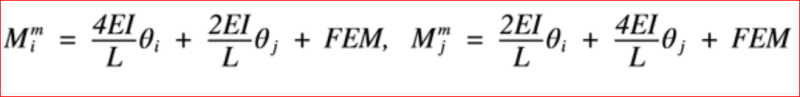Your sign convention indicates that clockwise rotation is negative, counter-clockwise positive, but you must include the FEM in the beam moment. If you do that, the sum of beam moments at each joint is zero.

BA

### RE: Structure Design Using Slope-Deflection Equations and Matlab

(OP)
Ah, a simple error. I left out the FEM. Its all good now.

You are right, changing linear equations to matrix form is easy. But for my larger structure it would be quite tedious putting the values into the matrix. So, for an even larger structures the equationsTomatrix function is very, very handy.

Thanks for all your help BAretired.

### RE: Structure Design Using Slope-Deflection Equations and Matlab

You're welcome, Tygra_1983.

BA

### RE: Structure Design Using Slope-Deflection Equations and Matlab

#### Quote (Tygra)

I have found a great function in Matlab called 'equationsToMatrix' that converts the linear equations into matrix form. Solving the matrix gave me the correct answers!
Congratulation Tygra! For MATLAB and structural analysis you would always want to take advantage of matrices as its basically {F}=[K]{u}. For your bigger structure, you can simplify things by having all FEM in one column vector matrix, slope equations in another matrix, and rotations in another matrix. With that you can easily eliminate joints of rows from matrices whose rotations are fixed.

#### Quote (BAretired)

Your sign convention indicates that clockwise rotation is negative, counter-clockwise positive,
I think Tygra is from Europe or UK. Remember to keep your signs and units consistent throughout the calculations. Wishing you all the best on your project!

### RE: Structure Design Using Slope-Deflection Equations and Matlab

You have to solve just two equations. the other equations automatically will be solve.

#### Red Flag This Post

Please let us know here why this post is inappropriate. Reasons such as off-topic, duplicates, flames, illegal, vulgar, or students posting their homework.

#### Red Flag Submitted

Thank you for helping keep Eng-Tips Forums free from inappropriate posts.
The Eng-Tips staff will check this out and take appropriate action.

#### Resources

Low-Volume Rapid Injection Molding With 3D Printed Molds
Learn methods and guidelines for using stereolithography (SLA) 3D printed molds in the injection molding process to lower costs and lead time. Discover how this hybrid manufacturing process enables on-demand mold fabrication to quickly produce small batches of thermoplastic parts. Download Now
Examine how the principles of DfAM upend many of the long-standing rules around manufacturability - allowing engineers and designers to place a partâ€™s function at the center of their design considerations. Download Now
Industry Perspective: Education and Metal 3D Printing
Metal 3D printing has rapidly emerged as a key technology in modern design and manufacturing, so itâ€™s critical educational institutions include it in their curricula to avoid leaving students at a disadvantage as they enter the workforce. Download Now

Close Box

# Join Eng-Tips® Today!

Join your peers on the Internet's largest technical engineering professional community.
It's easy to join and it's free.

Here's Why Members Love Eng-Tips Forums:

•Talk To Other Members
• Notification Of Responses To Questions
• Favorite Forums One Click Access
• Keyword Search Of All Posts, And More...

Register now while it's still free!